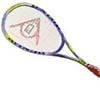#### You may also like### Rain or Shine

Predict future weather using the probability that tomorrow is wet given today is wet and the probability that tomorrow is wet given that today is dry.### Squash

If the score is 8-8 do I have more chance of winning if the winner is the first to reach 9 points or the first to reach 10 points?### Playing Squash

Playing squash involves lots of mathematics. This article explores the mathematics of a squash match and how a knowledge of probability could influence the choices you make.

# Ante Up

### Why do this problem?

This problem provides an interesting backdrop for computations in combinatorics and conditional probability. The results are very counter-intuitive to most people: although each sequence of three H/T combinations is equally likely, for any given sequence you can always choose another sequence which is likely to come first out of the Turing machine.

The problem will provide stimulation for wider background reading for students with an interest in probability and uncertainty.

### Possible approach

You can introduce the concept of the machine through a game with the interactivity: Students pair up and each choose a sequence of three heads or tails. Start the machine (with no choices in the interactivity, so that it doesn't stop) and then keep going until one of each pair has lost. Then make pairs out of the winners and repeat until a single champion is left.

Now pose the question for the first part of the problem, in which there are 6 units of paper left in the machine: "Who do we think is more likely to win?". Regardless of the answers given, we need to then ask the question "How do you know?". The only sound answer to this is through direct computation.

Drawing a tree is a sensible way forward, as is enumerating all of the $2^6$ possibilities and seeing who will be the winner in each individual case. However, students should be encouraged to draw out trees cleverly 'one letter at a time', as when either Turing or I win there is no point continuing that branch of the tree. Note that the question does not directly ask for the probability of a winner, merely which is more likely to win - students might be able to use this idea cleverly to save time on the computation. You can ask students who finish this quickly to work out the probabilities of a win or to consider the extension to 8 addition units of paper.

The second part of the problem flows naturally from the first part: however, here students will have to guess a string and compute the probabilities. Clear recording and teamwork might help here.

For the extension task, a tree diagram approach is a likely starting point but students will soon realise that it is impossible to draw out the 'complete tree' as  there is the possibility that the game will go on arbitrarily long without a winner. This is a good way to introduce ideas of recursive thinking in conditional probability and should be stimulating thinking for the most able students.

### Key questions

How many possible strips of 6 letters might emerge from the machine?
If at any time HH has just emerged from the machine, why is Turing GUARANTEED not to lose?
How might a tree diagram help?
Is there a sensible way to list all of the possibilities of H/T combinations?

### Possible extension

There are two possible extensions:

1) Construct a table with all of the probabilities of a victory for me for all choices for me and Turing, given that there are 8 units in the machine to begin with.
2) There is an interesting article on this and other related concepts at http://plus.maths.org/issue55/features/nishiyama/. As an extension, very interested students could work through the calculations and verify some of the probabilities.

### Possible support

You could simplify matters by suggesting that the machine initially only holds 4 letters -- this will make drawing the complete tree diagram much simpler, and the task is then to determine which branch leads to a win for either Turing or myself.

You can also make use of this spreadsheet to work out the wins and losses.